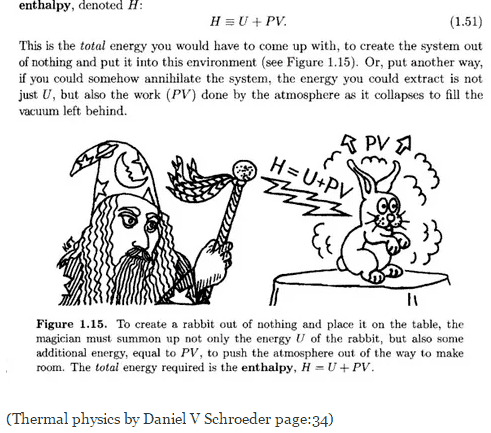# Enthalpy and mass energy

Hi,

I have been trying to understand what is enthalpy (H = U + PV). What has been my current understanding is it is the energy content in a system. And this nicely brings together the energy contents in terms of the 3 state variables, Temperature, Pressure and Volume.

If enthalpy is the energy content of the system. ie, the energy required to create the system given its Temperature, Volume and Pressure. Why is energy contained in the mass of the system (E= mc^2) not considered here ? Shouldn't it be H = U + PV + mc^2 ?

I have been trying to understand what is enthalpy (H = U + PV). What has been my current understanding is it is the energy content in a system.

The energy content of the system is the internal energy U. The enthalpy has been designed for better experimental handling. According to the first law of thermodynamics the change of internal energy of a closed system is the sum of work and heat exchanged with the environment. With limitation to volumetric work this means

dU = dq + dw = dq - p·dV

As it is very hard to keep the volume constant for solid or liquid systems this is a problem for calorimetric measurement because heat and volumetric work always need to be considered or measured simultaneously. That's were the enthalpy comes into play. The change of enthalpy is

dH = dU + p·dV + V·dp = dq + V·dp

Under isobaric conditions, which can easily be established (e.g. by open the system to atmospheric pressure), this is equal to the exchanged heat. That means the change of enthalpy can directly be determined with calorimetric measurements and the heat exchange can be calculated from a known enthalpy difference before and after the process.

If enthalpy is the energy content of the system. ie, the energy required to create the system given its Temperature, Volume and Pressure. Why is energy contained in the mass of the system (E= mc^2) not considered here ? Shouldn't it be H = U + PV + mc^2 ?

The rest energy mc² is included into the internal energy and they can usually be assumed to be identical.

•Ravi Singh choudhary, vjanandr and Chestermiller
jambaugh
Gold Member
Enthalpy is the energy of a system within a constant pressure environment. It adds to the internal energy U the energy required to displace the environmental fluid.

Well if you want to add in the mc^2 term then go ahead, it is simply a change of zero energy in the non-relativistic setting which, being constant will have no effect on the physics. Typically mass-energy conversion won't be occurring in an equilibrium setting if the substance is a simple gas.

If you actually want to carry out a relativistic treatment of the system then "you're going to need a bigger boat".

•vjanandr
Chestermiller
Mentor
The energy content of the system is the internal energy U. The enthalpy has been designed for better experimental handling. According to the first law of thermodynamics the change of internal energy of a closed system is the sum of work and heat exchanged with the environment. With limitation to volumetric work this means

dU = dq + dw = dq - p·dV

As it is very hard to keep the volume constant for solid or liquid systems this is a problem for calorimetric measurement because heat and volumetric work always need to be considered or measured simultaneously. That's were the enthalpy comes into play. The change of enthalpy is

dH = dU + p·dV + V·dp = dq + V·dp

Under isobaric conditions, which can easily be established (e.g. by open the system to atmospheric pressure), this is equal to the exchanged heat. That means the change of enthalpy can directly be determined with calorimetric measurements and the heat exchange can be calculated from a known enthalpy difference before and after the process.

The rest energy mc² is included into the internal energy and they can usually be assumed to be identical.
I strongly agree with, and relate to, this explanation. I have never seen a satisfactory (to me) physical interpretation of enthalpy (and, in my judgment, none is needed). As far as I'm concerned, enthalpy is just a very convenient property (thermodynamic function) to work with in describing the thermodynamic behavior of many different situations.

Chet

The rest energy mc² is included into the internal energy and they can usually be assumed to be identical.
but isn't the internal energy attributed to the Kinetic energy possessed by the atoms by virtue of the temperature or heat energy ?, so shouldn't the internal energy be zero at 0 kelvin. Therefore internal energy shouldn't contain the rest energy which is independent of temperature or kinetic energy of the atoms ? Its the energy by virtue of its mass and not by its motion ?

This question arised from the description of enthalpy here https://www.quora.com/What-are-the-intuitive-explanations-of-internal-energy-and-enthalpy
I am attaching the snipped that the author had presented there.I felt to create the system, we first need energy to create matter itself and that is not being considered.

but isn't the internal energy attributed to the Kinetic energy possessed by the atoms by virtue of the temperature or heat energy ?

Internal energy is the sum of all energies of a system except the kinetic energy of the whole system and its potential energy in external fields. That means it consists not only of the thermal energy but of any kind of energy within the system including internal potential energy, rest energies of particles and even vacuum energy.

•vjanandr